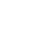Live chatHello Carl;

## Ref: Managerial Accounting Practice IP5

Following your email on the operations you have undertaken in your company, I am glad to take this opportunity to analyze California Container division’s breakeven point. The company produces packaging for cell phone; therefore its calculations will be as follows:

a) Old break even quantity

Sales   = \$3.24 per unit

V.C      = \$1.37 per unit

F.C      = \$257,000

Sales = Variable Costs (VC) + Fixed expenses (FC) + Profit

Let Quantity be Q;

\$3.24Q = \$1.37Q + \$127,000 + \$0

\$1.87Q = \$127,000

Q         = 67, 915 units

b)  New Break even quantity

Sales    = \$3.24 per unit

V.C     = \$1.52 per unit

F.C      = \$257,000

Sales = Variable Costs (VC) + Fixed expenses (FC) + Profit

Let Quantity be Q;

\$3.24Q = \$1.52Q + \$127,000 + \$0

\$1.72Q = \$127,000

Q         = 73,837 unitsc)  Sales = Variable Costs (VC) + Fixed expenses (FC) + Profit

Old profit = Sales – VC –FC= (3,300,000 * 3.24) – (1.37 * 3,300,000) – 257,000= \$5,914,000

For the same profit to be realized, due to increase in variable costs, number of packages need to be increased.

Profit = Sales – VC – FC

\$5,914,000 = \$3.24Q -\$1.52Q - \$127,000

\$1.72Q = \$6,041,000

Q = 3,512,210 packages

Therefore the company needs 212,210 (3,512,210 – 3,300,000) more packages in order to realize the same profit.

0

Preparing Orders

0

Active Writers

0%

Positive Feedback

0

Support Agents

Limited offerget 15% off your 1st order with code first15